# ISRO Scientist or Engineer Electronics 2010

Instructions

For the following questions answer them individually

Question 71

# Theelectric field intensity at a distance of 10km from an antenna having a directive gain of 10dB andradiating a total power of 60kW isQuestion 72

# What is the characteristic impedance of a coaxial transmission line having inner conductor radius of Imm, outer conductor radius of 2mm anddielectric constant of 4.0?Question 73

# Animpedance of -10 — j2 ohmsis connected to another impedance of 45 + j5 ohms through a transmission line having a characteristic impedance of 50 ohms. Assess the stability of this circuitQuestion 74

# A transmission line of characteristic impedance 75 ohms is terminated with an impedance of 50 ohms. The line length is increased from zero. What will be the locus of the input impedance at the other end of the line? (N.B. : Reference impedance of Smith Chart = 50 Ohms)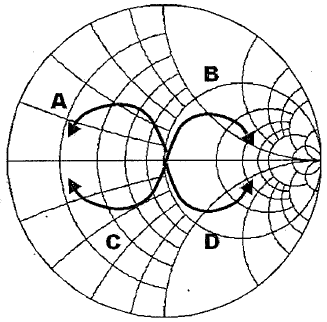Question 75

# The value of the integral $$\int \frac{\sin Z}{Z^{3}} .dz$$ around the circle $$\mid Z \mid = 2$$ isQuestion 76

# Which of the following functions have a jump discontinuity at x = 0Question 77

# What would be the output of the following circuit, if a positive going unipolar pulse with an amplitude greater than $$V_ref$$, is applied at the input?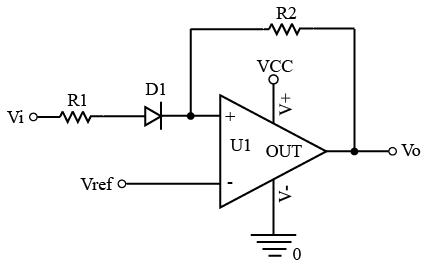Question 78

# What is the output voltage across the 900 ohm load in the circuit given below?.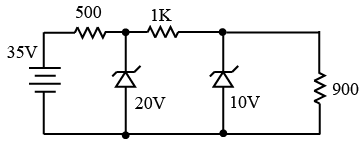Question 79

# What is the transfer function of the network given below?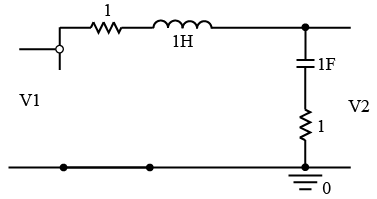Question 80

# A voltage regulator having $$V_{ref}$$=1.25V needs to generate 5V output. Assumethat $$I_{adj}$$ = 100nA and $$R_{1}$$ = 10kohm. What should be value of $$R_{2}$$?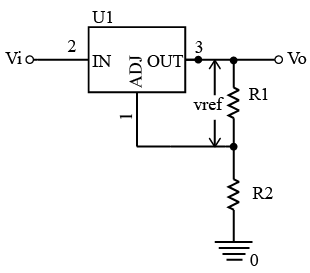OR